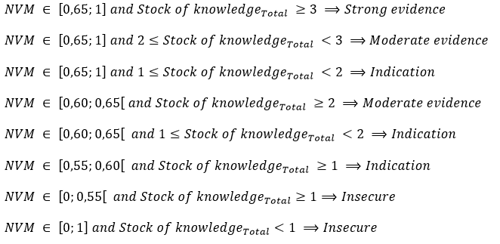# Calculations

### Calculation of the job effect and the level of evidence

On Jobeffekter.dk you can view the job effect for a given intervention and target group, and the level of evidence for the job effect. The following describes the calculation of the job effect and the level of evidence in six steps.

1. Step - Calculating the knowledge contribution

First, the knowledge contribution is calculated for all results concerning the intervention and the target group by using the weights of the study and the combination factor: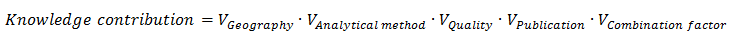The knowledge contribution describes the value that should be added to a result when calculating the job effect.  It expresses the impact of the individual result on the job effect. The knowledge contribution can be between 0 and 1.

2. Step - Calculating the stock of knowledge

The overall stock of knowledge for the job effect is then calculated by aggregating the calculated knowledge contributions: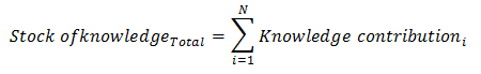The stock of knowledge describes the knowledge base underlying the job effect when taking into account the quality and relevance of the results. The stock of knowledge can, as a minimum, be 0 and, as a maximum, be equal to the number of results concerning the intervention and the target group.

If the stock of knowledge is less than 1, the overall conclusion on the effect will be "Few studies".

3. Step – Determining the dominant effect direction

The dominant effect direction is then selected by calculating and comparing the stock of knowledge for each of the possible effect directions (positive, negative and no effect). The stock of knowledge that supports a given effect direction is calculated as: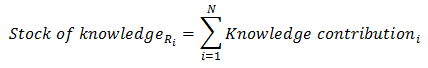Where Ri indicates the effect direction in result i, that is Ri {-1, 0, +1}

The stock of knowledge for each of the three effect directions is used to determine whether the dominant effect direction is negative, positive or has no effect. An effect direction is dominant if the stock of knowledge supporting the effect direction is greater than the stock of knowledge not supporting the effect direction. The dominant effect direction is therefore determined as follows: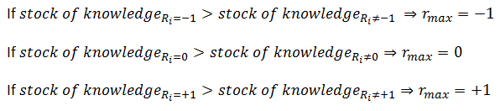Where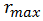indicates the dominant effect direction, and -1 corresponds to a negative effect, 0 corresponds to no effect and +1 corresponds to a positive effect.

If no dominant effect direction can be determined, the overall conclusion on effect will be “Conflicting".

4. Step – Calculating the relative net stock of knowledge

When the dominant effect direction has been determined, the relative net stock of knowledge (Danish abbreviation NVM - nettovidensmængde) is calculated by dividing the stock of knowledge supporting the dominant effect direction by the overall stock of knowledge: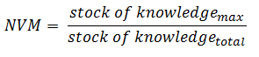The greater the percentage of the overall stock of knowledge supporting the dominant effect direction, the greater the relative net stock of knowledge. If all studies support the same effect direction, the value of the relative net stock of knowledge is 1.

If the net stock of knowledge is less than 0,55, the overall conclusion on the effect will be "Few studies" or "Conflicting".

5. Step – Determining the job effect

The job effect can be determined on the basis of the above.

The job effect is determined on the basis of whether the overall stock of knowledge and net stock of knowledge are greater or smaller than 1 and 0,55, respectively. The job effect equals the dominant effect direction, if the overall stock of knowledge is greater than 1 and the net stock of knowledge (NVM) is between 0,55 and 1. The job effect is therefore determined as follows: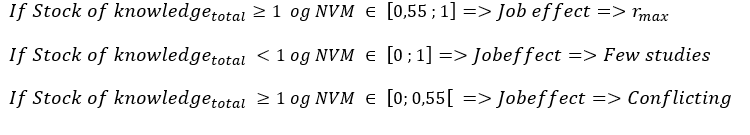Whereindicates the dominant effect direction which can be positive, negative or have no effect.

6. Step - Calculating the level of evidence

On the basis of the value of the relative net stock of knowledge, the level of evidence is assessed for the job effect. The level of evidence describes how robust the job effect is assessed to be, and the effect can have "Strong evidence", "Moderate evidence", be an "Indication" or "Uncertain".

The categorization of the level of evidence is based in the following intervals of the net stock of knowledge: